Author: Oscar Cronquist Article last updated on January 20, 2023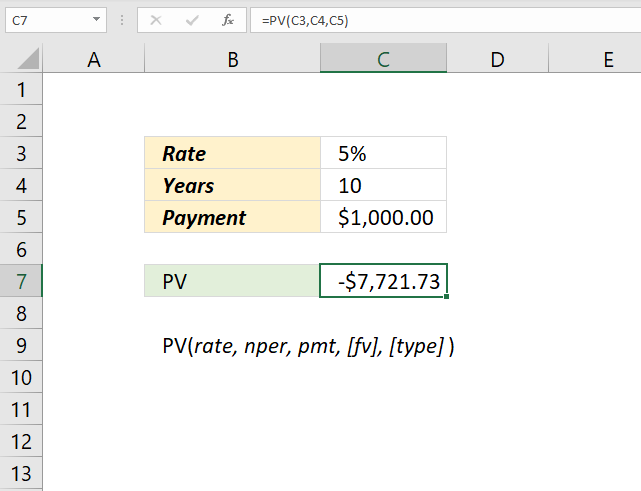The PV function calculates the net present value for an investment or loan. PV stands for present value.

## 1. PV Function Syntax

PV(rate, nper, pmt, [fv], [type])

## 2. PV Function Arguments

 rate Required. The interest rate per period. If nper is years then the interest rate is per year. Divide the interest rate with 12 if you use months in the nper argument. nper Required. The total number of payment periods in an annuity. pmt Required. The payment amount per period. Interest and principal is often included in this amount but not other fees or taxes. The pmt argument is not used if you use the [fv] argument. [fv] Optional. The future value. [type] Optional. The number 0 or 1 and indicates when payments are due.

## 3. PV Function exampleFormula in cell C7:

=PV(C3, C4, C5)

## 4. What is the formula behind the PV function?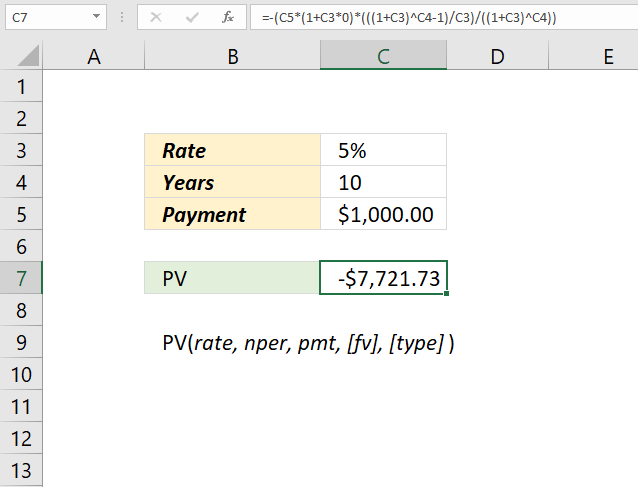Formula:

(-pmt(1+rate*type)*(((1+rate^nper)-1)/rate)-fv)/((1+rate)^nper)

Formula in cell C7:

=-(C5*(1+C3*0)*(((1+C3)^C4-1)/C3)/((1+C3)^C4))

## 5. How is the result of the PV function calculated in detail?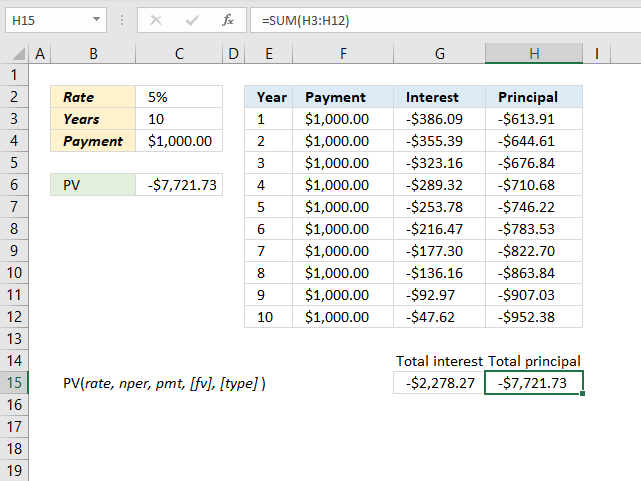The PV function in cell C6 calculates the present value of an annuity loan or the amount you can borrow when the interest rate is 5% per year and the loan is paid back in ten years. In other words, it calculates how much the loan is based on a given interest rate, how much you pay per period, and the amount you pay per period.

An annuity loan is paid back for a specific time range at a given interest rate with the same amount for all payments.

The table in cell range E2:H12 shows interest and principal for each period based on values in cell range C2:C4. The total principal (or amortization) paid is the same as the present value in cell C6, which is needed to pay back the entire loan.

Formula in cell C6:

=PV(C3, C4, C5)

The result in cell C6 is the present value of the loan based on the interest rate, periods, and payment amount.

The formula in cell G3 calculates the interest included in each payment.

Formula in cell G3:

=(\$C\$6-SUM(\$H\$2:H2))*\$C\$2

The principal is what's left after the interest is paid, it is calculated in cell h3.

Formula in cell H3:

=-(F3+G3)

The formulas in cell G15 and H15 adds the amounts and return the total of interest and principal respectively.

Formula in cell G15:

=SUM(G3:G12)

Formula in cell H15:

=SUM(H3:H12)

## 6. What anount is needed if I want x in future value, the interest rate is constant, and the number of periods is given?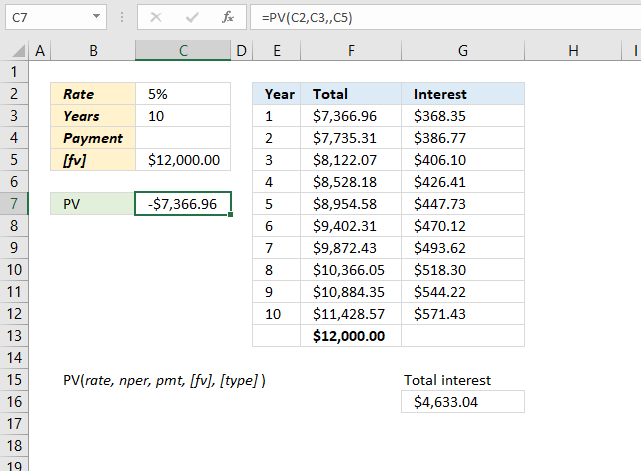The image above demonstrates how to use the PV function when you want to know the amount needed for an investment when the interest rate is specified, and the number of periods are given.

Formula in cell C7:

=PV(C2, C3,,C5)

Note that the pmt argument can be empty when you specify the fv (future value) argument in the PV function.

The table, shown in the image above, displays the start amount and the interest for each period. The total accumulates the interest (column F) and the fv (future value) is reached after the given period. The table is not needed for the calculation, it is only there to show that the numbers add up and are correct.

See cell F13, it is equal to the future value in cell C5.

Formula in cell F3:

=-\$C\$7+SUM(\$G\$2:G2)

Copy cell F3 and paste cells below.

Formula in cell G3:

=\$C\$2*F3

Copy cell G3 and paste cells below.

## 7. What amount is needed if I want x in future value, the interest rate is constant, the payment amount, and the number of periods are given?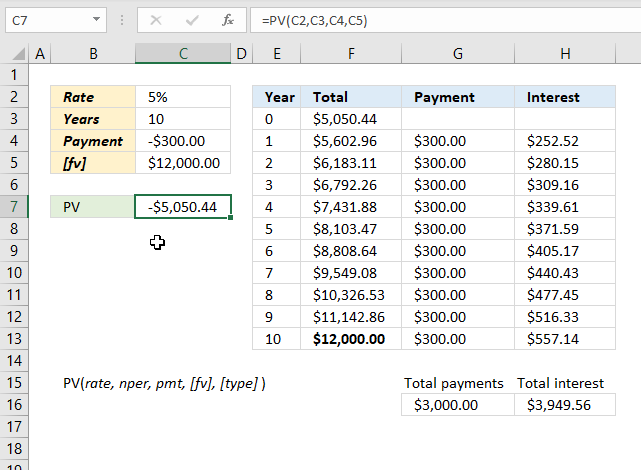The image above shows what amount is needed if you save x amount for each period to reach a future value [fv) given a constant interest rate.

Formula in cell C7:

=PV(C2, C3, C4, C5)

The table in cell range E2:H13 shows that the numbers add up to the future value, specified in cell C5, after the given time of periods based on a constant interest rate.

The table is not needed to calculate the present value of the investment, the PV function is all that is needed.

Formula in cell F3:

=-\$C\$7+SUM(\$H\$2:H3)+SUM(\$G\$2:G3)

Copy cell F3 and paste to cells below as far as needed.

Formula in cell G4:G13:

=-\$C\$4

Formula in cell H4:

=\$C\$2*F3

Copy cell H4 and paste to cells below as far as needed.